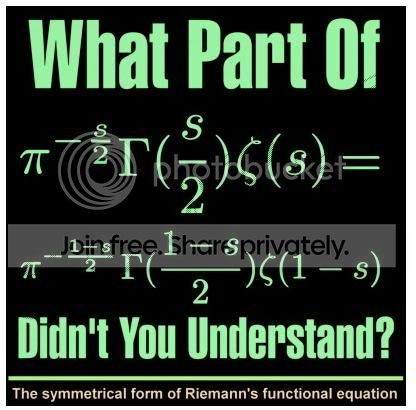Search IntMath
Close

# What part of … didn’t you understand?

By Murray Bourne, 10 Oct 2008

This is fun. A lot of mathematics looks like a foreign language, so the question in the image is reasonable:Image source

For some background (for the math nerds), see

Be the first to comment below.

### Comment Preview

HTML: You can use simple tags like <b>, <a href="...">, etc.

To enter math, you can can either:

1. Use simple calculator-like input in the following format (surround your math in backticks, or qq on tablet or phone):
a^2 = sqrt(b^2 + c^2)
(See more on ASCIIMath syntax); or
2. Use simple LaTeX in the following format. Surround your math with $$ and $$.
$$\int g dx = \sqrt{\frac{a}{b}}$$
(This is standard simple LaTeX.)

NOTE: You can mix both types of math entry in your comment.